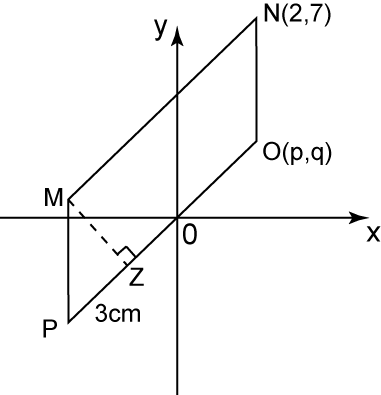# 9.3.4 Straight Lines, PT3 Focus Practice

Question 7:The diagram above shows a parallelogram on a Cartesian plane. MP and NO are parallel to the y-axis. Given that the distance of MZ is 4 units. Find
(a) the value of p and q.
(b) the equation of the straight line MN.

Solution:

Question 8:The diagram above shows that two straight line intersect at point (0 , -2). Find
(a) the value of b
(b) the x-intercept of the straight line XY if the gradient of XY is equal to 2.
(c) the equation of XY.

Solution:
(a)
Value of b
= 2 units + 3 units
= 5 units
= –5